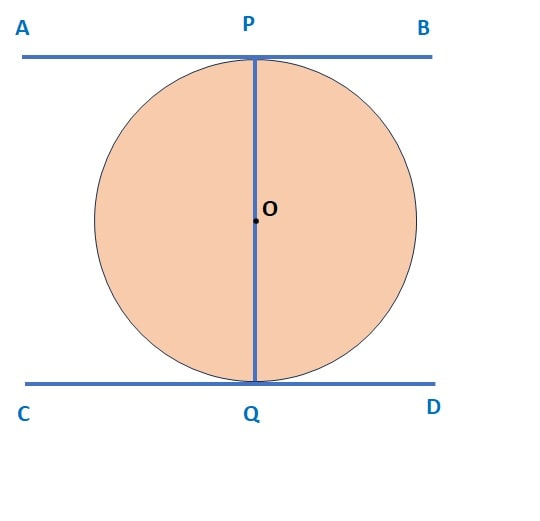Q) Prove that the tangents drawn at the ends of a diameter of a circle are parallel.Ans: Let AB and CD are the 2 lines.

Since, line AB is tangent to the circle at point P, therefore ∠ APO = 90° (angle between radius and tangent)

Similarly, line CD is tangent to the circle at point Q, therefore ∠ CQO = 90°

Now, in the above diagram, ∠ APO = 90° and ∠ CQO = 90°,

Since these two angles denote the interior angles on the same side of the transversal,

Therefore AB ǁ CD ………… Hence proved !

Scroll to Top